### Affordable pricing from £10/month

In this worksheet, students calculate angles in quadrilaterals.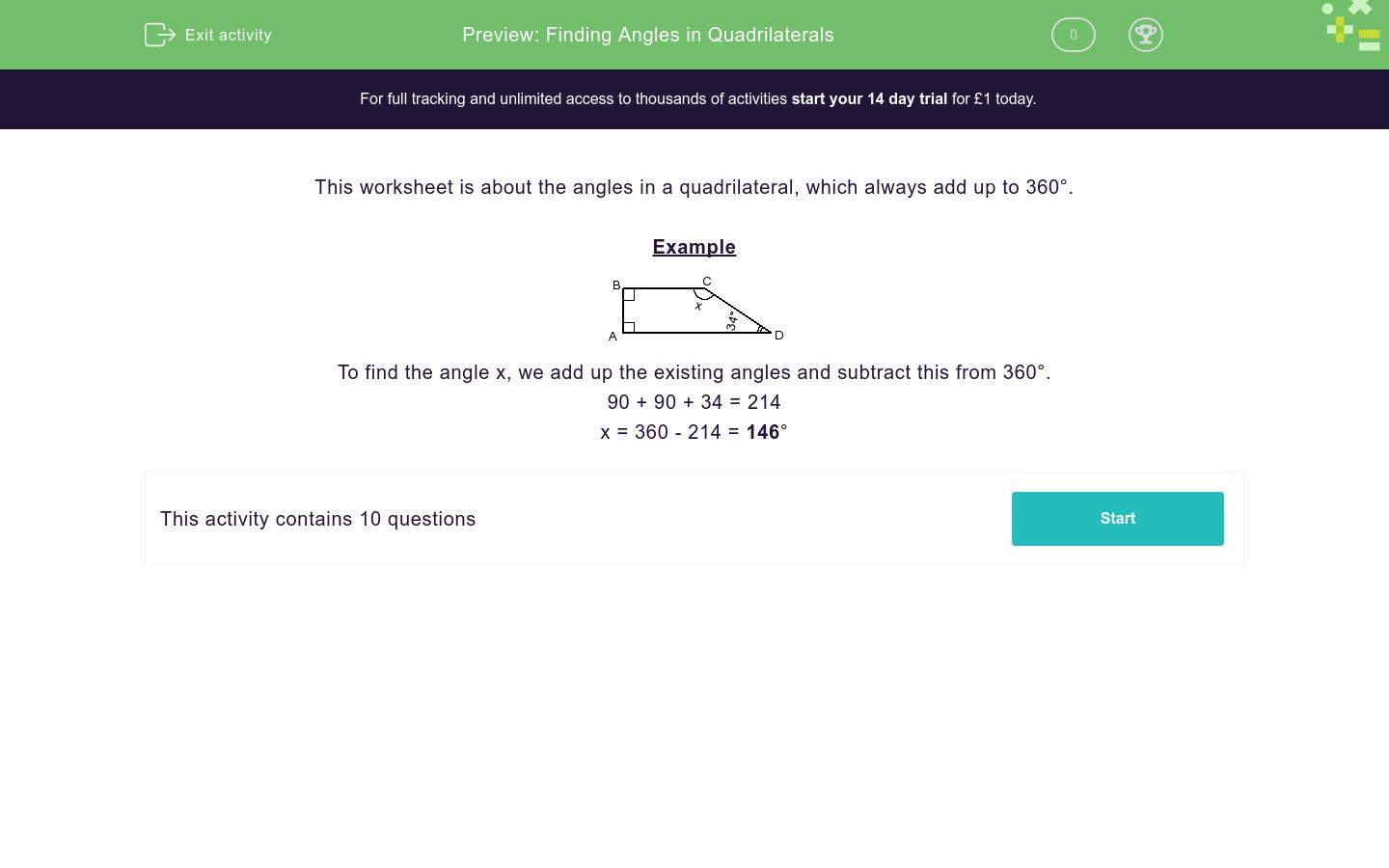Key stage:  KS 3

Curriculum topic:   Geometry and Measures

Curriculum subtopic:   Derive/Use the Sum of Angles for Deduction

Difficulty level:### QUESTION 1 of 10

Example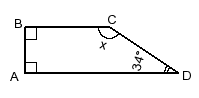To find the angle x, we add up the existing angles and subtract this from 360°.

90 + 90 + 34 = 214

x = 360 - 214 = 146°

Find the angle marked x in the following diagram.Find the angle marked x in the following diagram.Find the angle marked x in the following diagram.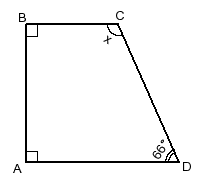Find the angle marked x in the following diagram.Find the angle marked x in the following diagram.Find the angle marked x in the following diagram.Find the angle marked x in the following diagram.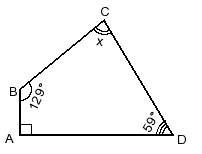Find the angle marked x in the following diagram.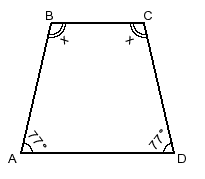Find the angle marked x in the following diagram.Find the angle marked x in the following diagram.• Question 1

Find the angle marked x in the following diagram.132
EDDIE SAYS
90 + 90 + 48 = 228 x = 360 - 228 = 132°
• Question 2

Find the angle marked x in the following diagram.106
EDDIE SAYS
90 + 94 + 70 = 254 x = 360 - 254 = 106°
• Question 3

Find the angle marked x in the following diagram.114
EDDIE SAYS
90 + 90 + 66 = 246 x = 360 - 246 = 114°
• Question 4

Find the angle marked x in the following diagram.116
EDDIE SAYS
90 + 98 + 56 = 244 x = 360 - 244 = 116°
• Question 5

Find the angle marked x in the following diagram.85
EDDIE SAYS
72 + 126 + 77 = 275 x = 360 - 275 = 85°
• Question 6

Find the angle marked x in the following diagram.85
EDDIE SAYS
90 + 103 + 82 = 275 x = 360 - 275 = 85°
• Question 7

Find the angle marked x in the following diagram.82
EDDIE SAYS
90 + 129 + 59 = 278 x = 360 - 278 = 82°
• Question 8

Find the angle marked x in the following diagram.103
EDDIE SAYS
77 + 77 = 154 2x = 360 - 154 = 206 x = 103°
• Question 9

Find the angle marked x in the following diagram.146
EDDIE SAYS
90 + 129 + 34 = 214 x = 360 - 214 = 146°
• Question 10

Find the angle marked x in the following diagram.75
EDDIE SAYS
45 + 177 + 63 = 285 x = 360 - 285 = 75°
---- OR ----

Sign up for a £1 trial so you can track and measure your child's progress on this activity.

### What is EdPlace?

We're your National Curriculum aligned online education content provider helping each child succeed in English, maths and science from year 1 to GCSE. With an EdPlace account you’ll be able to track and measure progress, helping each child achieve their best. We build confidence and attainment by personalising each child’s learning at a level that suits them.

Get started